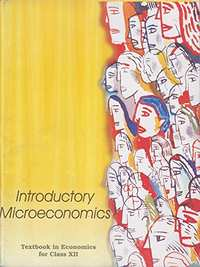Share

# NCERT solutions for Class 12 Economics - Introductory Microeconomics chapter 6 - Non-Competitive Markets [Latest edition]

Textbook page

#### Chapters## Chapter 6: Non-Competitive Markets

Exercise

#### NCERT solutions for Class 12 Economics - Introductory Microeconomics Chapter 6 Non-Competitive Markets Exercise [Pages 87 - 101]

Exercise | Q 1 | Page 100

What would be the shape of the demand curve so that the total revenue curve is

(a) a positively sloped straight line passing through the origin?

(b) a horizontal line?

Exercise | Q 2 | Page 100

From the schedule provided below calculate the total revenue, demand curve and the price elasticity of demand:

 Quantity 1 2 3 4 5 6 7 8 9 Marginal Revenue 10 6 2 2 2 0 0 0 −5
Exercise | Q 3 | Page 100

What is the value of the MR when the demand curve is elastic?

Exercise | Q 4 | Page 101

A monopoly firm has a total fixed cost of Rs 100 and has the following demand schedule:

 Quantity 1 2 3 4 5 6 7 8 9 10 Price 100 90 80 70 60 50 40 30 20 10

Findthe short run equilibrium quantity, price and total profit. What would be the equilibrium in the long run? In case the total cost is Rs 1000, describe the equilibrium in the short run and in the long run.

Exercise | Q 5 | Page 101

If the monopolist firm of Exercise 3, was a public sector firm. The government set a rule for its manager to accept the government fixed price as given (i.e. to be a price taker and therefore behave as a firm in a perfectly competitive market). And the government decide to set the price so that demand and supply in the market are equal. What would be the equilibrium price, quantity and profit in this case?

Exercise | Q 6.1 | Page 101

Comment on the shape of MR curve in case when TR curve is a positively sloped straight line.

Exercise | Q 6.2 | Page 87

Comment on the shape of MR curve in case when TR curve is a horizontal straight line.

Exercise | Q 7 | Page 101

The market demand curve for a commodity and the total cost for a monopoly firm producing the commodity is given in the schedules below.

 Quantity 0 1 2 3 4 5 6 7 8 Price 52 44 37 3 26 22 19 16 13

 Quantity 0 1 2 3 4 5 6 7 8 Total Cost 10 60 90 100 102 105 109 115 125

Use the information given to calculate the following:

(a) The MR and MC schedules

(b) The quantities for which MR and MC are equal

(c) The equilibrium quantity of output and the equilibrium price of the commodity

(d) The total revenue, total cost and total profit in the equilibrium

Exercise | Q 8 | Page 101

Will the monopolist firm continue to produce in the short run if a loss is incurred at the best short run level of output?

Exercise | Q 9 | Page 101

Explain why the demand curve facing a firm under monopolistic competition is negatively sloped.

Exercise | Q 10 | Page 101

What is the reason for the long run equilibrium of a firm in monopolistic competition to be associated with zero profit?

Exercise | Q 11 | Page 101

List the three different ways in which oligopoly firms may behave.

Exercise | Q 12 | Page 101

If duopoly behaviour is one that is described by Cournot, the market demand curve is given by the equation q = 200 − 4p and both the firms have zero costs, find the quantity supplied by each firm in equilibrium and the equilibrium market price.

Exercise | Q 13 | Page 101

What is meant by prices being rigid? How can oligopoly behaviour lead to such an outcome?

## Chapter 6: Non-Competitive Markets

Exercise## NCERT solutions for Class 12 Economics - Introductory Microeconomics chapter 6 - Non-Competitive Markets

NCERT solutions for Class 12 Economics - Introductory Microeconomics chapter 6 (Non-Competitive Markets) include all questions with solution and detail explanation. This will clear students doubts about any question and improve application skills while preparing for board exams. The detailed, step-by-step solutions will help you understand the concepts better and clear your confusions, if any. Shaalaa.com has the CBSE Class 12 Economics - Introductory Microeconomics solutions in a manner that help students grasp basic concepts better and faster.

Further, we at Shaalaa.com provide such solutions so that students can prepare for written exams. NCERT textbook solutions can be a core help for self-study and acts as a perfect self-help guidance for students.

Concepts covered in Class 12 Economics - Introductory Microeconomics chapter 6 Non-Competitive Markets are Simple Monopoly in the Commodity Market, Other Non - Perfectly Competitive Markets, Simple Monopoly in the Commodity Market, Other Non - Perfectly Competitive Markets.

Using NCERT Class 12 solutions Non-Competitive Markets exercise by students are an easy way to prepare for the exams, as they involve solutions arranged chapter-wise also page wise. The questions involved in NCERT Solutions are important questions that can be asked in the final exam. Maximum students of CBSE Class 12 prefer NCERT Textbook Solutions to score more in exam.

Get the free view of chapter 6 Non-Competitive Markets Class 12 extra questions for Class 12 Economics - Introductory Microeconomics and can use Shaalaa.com to keep it handy for your exam preparation

S